Courses

# Practice Doc: CAT 2019 For Quant (Slot - 1) Notes | EduRev

## CAT : Practice Doc: CAT 2019 For Quant (Slot - 1) Notes | EduRev

The document Practice Doc: CAT 2019 For Quant (Slot - 1) Notes | EduRev is a part of CAT category.
All you need of CAT at this link: CAT

Q.1. Two cars travel the same distance starting at 10:00 am and 11:00 am, respectively, on the same day. They reach their common destination at the same point of time. If the first car travelled for at least 6 hours, then the highest possible value of the percentage by which the speed of the second car could exceed that of the first car is
(a) 20
(b) 30
(c) 25
(d) 10
Ans: a
Solution: Let the speed of cars be a and b and the distance = d
Minimum time taken by 1st car = 6 hours,
For maximum difference in time taken by both of them, car 1 has to start at 10:00 AM and car 2 has to start at 11 : 00 AM.
Hence, car 2 will take 5 hours.
Hence a = d/6 and b = d/5
Hence the speed of car 2 will exceed the speed of car 1 by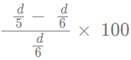d/30/d/6 x 100 = 20

Q.2. If a1, a2, ...... are in A.P., then,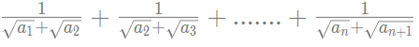is equal to
(a)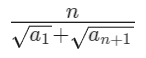(b)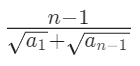(c)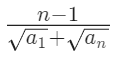(d)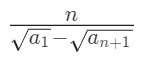Ans: a
Solution: We have,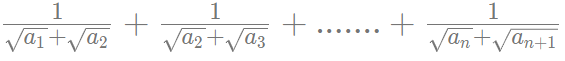Now,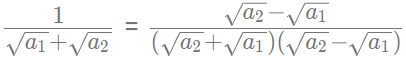(Multiplying numerator and denominator by √a2 - √a1)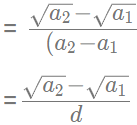(where d is the common difference)
Similarly,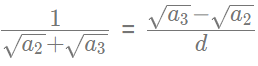and so on.
Then the expression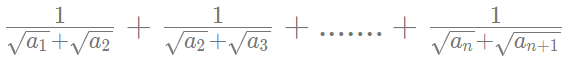can be written as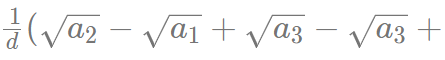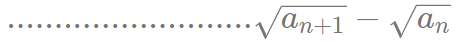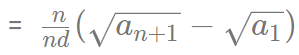(Multiplying both numerator and denominator by n)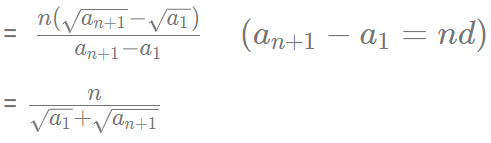Q.3. AB is a diameter of a circle of radius 5 cm. Let P and Q be two points on the circle so that the length of PB is 6 cm, and the length of AP is twice that of AQ. Then the length, in cm, of QB is nearest to
(a) 9.3
(b) 7.8
(c) 9.1
(d) 8.5
Ans:
c
Solution: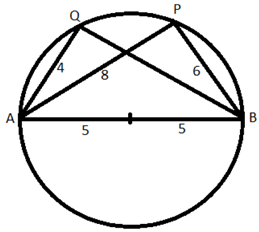Since AB is a diameter,  AQB and APB will right angles.
In right triangle APB, AP =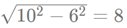Now, 2AQ = AP  => AQ= 8/2 = 4
In right triangle AQB, AP =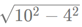= 9.165 = 9.1 (Approx)

Q.4. If (5.55)x = (0.555)y = 1000, then the value of 1/x 1/y is
(a) 1/3
(b) 3
(c) 1
(d) 2/3
Ans:
a
Solution: We have, (5.55)x = (0.555)= 1000,
x(log10555-2) = y(log10 555-3) = 3
Then, x(log10 555-2) = 3...(1)
y(log10555-3) = 3....(2)
From (1) and (2)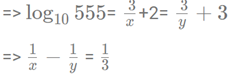Q.5. The income of Amala is 20% more than that of Bimala and 20% less than that of Kamala. If Kamala's income goes down by 4% and Bimala's goes up by 10%, then the percentage by which Kamala's income would exceed Bimala's is nearest to
(a) 31
(b) 29
(c) 28
(d) 32
Ans: a
Solution: Assuming the income of Bimla = 100a, then the income of Amala will be 120a.
And the income of Kamala will be 120a*100/80 = 150a
If Kamala's income goes down by 4%, then new income of Kamala = 150a - 150a(4/100) = 150a - 6a = 144a

If Bimla's income goes up by 10 percent, her new income will be 100a + 100a(10/100) = 110a
=> Hence the Kamala income will exceed Bimla income by (144a - 110a)*100/110a = 31

Q.6. The wheels of bicycles A and B have radii 30 cm and 40 cm, respectively. While traveling a certain distance, each wheel of A required 5000 more revolutions than each wheel of B. If bicycle B traveled this distance in 45 minutes, then its speed, in km per hour, was
(a) 18π
(b) 14π
(c) 16π
(d) 12π
Ans: c
Solution: Distance covered by A in 1 revolution = 2π * 30 = 60π
Distance covered by B in 1 revolution = 2π * 40 = 80π
Now, (5000 + n) 60π = 80π n
=> 15000 = 4n - 3n  =>n = 15000
Then distance travelled by B = 15000 * 80π  cm = 12π km
Hence, the speed =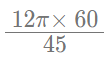= 16π

Q.7. The product of the distinct roots of |x- x - 6| = x + 2 is
(a) - 16
(b) - 4
(c) - 24
(d) - 8
Ans: a
Solution: We have, |x- x - 6| = x + 2
=> |(x - 3)(x + 2)| = x + 2
For x<-2, (3 - x)(-x - 2) = x + 2
=> x - 3 = 1   =>x = 4 (Rejected as x <- 2)
For -2 x<3, (3 - x)(x + 2) = x + 2 =>x = 2, -2
For x ≥ 3, (x - 3)(x + 2) = x + 2 => x = 4
Hence the product = 4*-2*2 = -16

Q.8. In a race of three horses, the first beat the second by 11 metres and the third by 90 metres. If the second beat the third by 80 metres, what was the length, in metres, of the racecourse?
Ans: 880
Solution: Assuming the length of race course = x and the speed of three horses be a, b and c respectively.
Hence,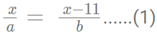and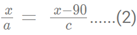Also,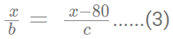From 1 and 2, we get,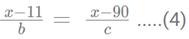Dividing (3) by (4), we get,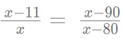=> (x - 11)(x - 80)= x(x - 90)
=> 91x - 90x = 880  => x = 880

Q.9. If the population of a town is p in the beginning of any year then it becomes 3 + 2p in the beginning of the next year. If the population in the beginning of 2019 is 1000, then the population in the beginning of 2034 will be
(a) (1003)15 + 6
(b) (997)15 − 3
(c) (997)214 + 3
(d) (1003)215 − 3
Ans: d
Solution: The population of town at the beginning of 1st year = p
The population of town at the beginning of 2nd year = 3 + 2p
The population of town at the beginning of 3rd year = 2(3 + 2p)+3 = 2 * 2p + 2*3 + 3 = 4p + 3(1 + 2)
The population of town at the beginning of 4th year = 2(2 * 2p + 2* 3 + 3) + 3 = 8p + 3(1 + 2 + 4)
Similarly population at the beginning of the nth year = 2n-1p+3(2n-1 - 1) = 2n-1 (p + 3)-3
The population in the beginning of 2019 is 1000, then the population in the beginning of 2034 will be (22034−2019) (1000 + 3)-3 = 215 (1003)-3

Q.10. Consider a function f satisfying f (x + y) = f (x) f (y) where x, y are positive integers, and f(1) = 2. If f(a + 1) +f (a + 2) +... + f(a + n) = 16 (2n - 1) then a is equal to
Ans: 3
Solution: f (x + y) = f (x) f (y)
Hence, f(2) = f(1 + 1) = f(1) * f(1)= 2 * 2 = 4
f(3) = f(2 + 1) = f(2) * f(1) = 4 * 2 = 8
f(4) = f(3 + 1) = f(3) * f(1) = 8 * 2 = 16
...... => f(x) = 2x
Now, f(a + 1) +f (a + 2) + ... + f(a + n) = 16 (2n - 1)
On putting n = 1 in the equation we get, f(a + 1) = 16
=> f(a)*f(1) = 16  (It is given that f (x + y) = f (x) f (y))
=> 2a* 2 = 16
=> a = 3

Q.11. Amala, Bina, and Gouri invest money in the ratio 3 : 4 : 5 in fixed deposits having respective annual interest rates in the ratio 6 : 5 : 4. What is their total interest income (in Rs) after a year, if Bina's interest income exceeds Amala's by Rs 250?
(a) 6350
(b) 6000
(c) 7000
(d) 7250
Ans: d
Solution: Assuming the investment of Amala, Bina, and Gouri be 300x, 400x and 500x, hence the interest incomes will be 300x*6/100=18x, 400x*5/100=20x and 500x*4/100 = 20x
Given, Bina's interest income exceeds Amala by 20x - 18x = 2x = 250  => x = 125
Now, total interest income = 18x + 20x + 20x = 58x = 58*125 = 7250

Q.12. For any positive integer n, let f(n) = n(n + 1) if n is even, and f(n) = n + 3 if n is odd. If m is a positive integer such that 8f(m + 1) - f(m) = 2, then m equals
Ans: 10
Solution: Assuming m is even, then 8f(m + 1) - f(m) = 2
m + 1 will be odd
So, 8(m + 1 + 3) -m(m + 1) = 2
=> 8m + 32-m2 - m =2
=> m2 − 7m − 30 = 0
=> m = 10, -3
Rejecting the negative value, we get m = 10
Assuming m is odd, m + 1 will be even.
then, 8(m + 1)(m + 2)-m-3 = 2
=> 8(m2 + 3m + 2)-m-3 = 2
=> 8m2 + 23m + 11 = 0
Discriminant for the equation = 232 - 4 • 8 • 11 = 177
Hence, the value of m will not be integral. Hence this case will be rejected.

Q.13. The product of two positive numbers is 616. If the ratio of the difference of their cubes to the cube of their difference is 157 : 3, then the sum of the two numbers is
(a) 58
(b) 85
(c) 50
(d) 95
Ans: c
Solution: Assume the numbers are a and b, then ab = 616
We have,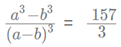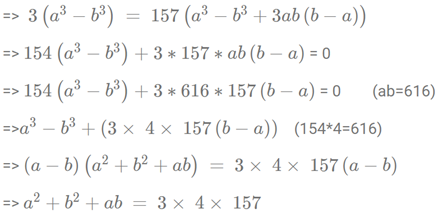Adding ab = 616 on both sides, we get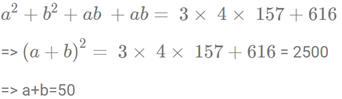Q.14. One can use three different transports which move at 10, 20, and 30 kmph, respectively to reach from A to B. Amal took each mode of transport for 1/3rd of his total journey time, while Bimal took each mode of transport for 1/3rd of the total distance. The percentage by which Bimal’s travel time exceeds Amal’s travel time is nearest to
(a) 22
(b) 20
(c) 19
(d) 21
Ans: a
Solution: Assume the total distance between A and B as d and time taken by Amal = t
Since Amal travelled 1/3rd of his total journey time in different speeds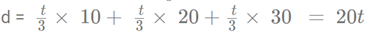Total time taken by Bimal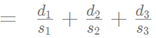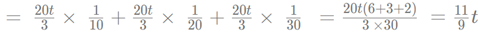Hence, the ratio of time taken by Bimal to time taken by Amal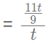= 11/9
Therefore, Bimal will exceed Amal's time by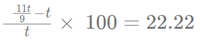Q.15. Meena scores 40% in an examination and after review, even though her score is increased by 50%, she fails by 35 marks. If her post-review score is increased by 20%, she will have 7 marks more than the passing score. The percentage score needed for passing the examination is
(a) 60
(b) 80
(c) 70
(d) 75
Ans: c
Solution: Assuming the maximum marks =100a, then Meena got 40a
After increasing her score by 50%, she will get 40a(1 + 50/100) = 60a
Passing score = 60a + 35
Post review score after 20% increase = 60a*1.2 = 72a
=>Hence, 60a + 35 + 7 = 72a
=>12a = 42   =>a = 3.5
=> maximum marks = 350 and passing marks = 210 + 35 = 245
=> Passing percentage = 245*100/350 = 70

Q.16. A person invested a total amount of Rs 15 lakh. A part of it was invested in a fixed deposit earning 6% annual interest, and the remaining amount was invested in two other deposits in the ratio 2 : 1, earning annual interest at the rates of 4% and 3%, respectively. If the total annual interest income is Rs 76000 then the amount (in Rs lakh) invested in the fixed deposit was
Ans: 9
Solution: Assuming the amount invested in the ratio 2 : 1 was 200x and 100x, then the fixed deposit investment = 1500000 - 300x
Hence, the interest = 200x*4/100 = 8x and 100x*3/100 = 3x
Interest from the fixed deposit = (1500000-300x)*6/100 = 90000 - 18x
Hence the total interest  = 90000 - 18x + 8x + 3x = 90000 - 7x = 76000
=> 7x = 14000   => x = 2000
Hence, the fixed deposit investment = 1500000 - 300*2000 = 900000 = 9 lakhs

Q.17. A club has 256 members of whom 144 can play football, 123 can play tennis, and 132 can play cricket. Moreover, 58 members can play both football and tennis, 25 can play both cricket and tennis, while 63 can play both football and cricket. If every member can play at least one game, then the number of members who can play only tennis is
(a) 38
(b) 32
(c) 45
(d) 43
Ans: d
Solution: Assume the number of members who can play exactly 1 game = I
The number of members who can play exactly 1 game = II
The number of members who can play exactly 1 game = III
I + 2II + 3III = 144 + 123 + 132 = 399....(1)
I + II + III = 256......(2)
=> II + 2III = 143.....(3)
Also, II + 3III = 58 + 25 + 63 = 146 ......(4)
=> III = 3 (From 3 and 4)
=> II = 137
=> I = 116
The members who play only tennis = 123 - 58 - 25 + 3 = 43

Q.18. If a+ a2 + a3 + .... + an = 3(2n +1 - 2), for every n ≥ 1, then a11 equals
Ans: 6144
Solution: 11th term of series = a11 Sum of 11 terms - Sum of 10 terms = 3(211+1 - 2)-3 (210+1 - 2)
= 3 (212 - 2 - 211 + 2)=3(211)(2 - 1) = 3*211 = 6144

Q.19. The number of the real roots of the equation 2Cos(x(x + 1)) = 2x + 2-x is
(a) 2
(b) 1
(c) infinite
(d) 0
Ans: b
Solution: 2 cos(x(x + 1)) = 2x + 2−x
The maximum value of LHS is 2 when cos(x(x + 1)) is 1 and the minimum value of RHS is 2 using AM ≥ GM
Hence LHS and RHS can only be equal when both sides are 2. For LHS, cosx(x + 1) = 1   => x(x + 1) = 0   => x = 0,-1
For RHS minimum value, x = 0
Hence only one solution x = 0

Q.20. At their usual efficiency levels, A and B together finish a task in 12 days. If A had worked half as efficiently as she usually does, and B had worked thrice as efficiently as he usually does, the task would have been completed in 9 days. How many days would A take to finish the task if she works alone at her usual efficiency?
(a) 36
(b) 24
(c) 18
(d) 12
Ans: c
Solution: Assuming A completes a units of work in a day and B completes B units of work in a day and the total work = 1 unit
Hence, 12(a + b) = 1.........(1)
Also, 9(a/2 + 3b) =1  .........(2)
Using both equations, we get, 12(a + b) = 9 (a/2 + 3b)
=> 4a + 4b = 3a/2 + 9b
=> 5a/2 = 5b
=> a = 2b
Substituting the value of b in equation (1),
12(3a/2) = 1
=> a = 1/18
Hence, the number of days required = 1/(1/18) = 18

Q.21. In a class, 60% of the students are girls and the rest are boys. There are 30 more girls than boys. If 68% of the students, including 30 boys, pass an examination, the percentage of the girls who do not pass is
Ans: 20
Solution: Assuming the number of students =100x
Hence, the number of girls = 60x and the number of boys = 40x
We have, 60x - 40x = 30  => x = 1.5
The number of girls = 60*1.5 = 90
Number of girls that pass = 68x - 30 = 68*1.5 - 30 = 102 - 30 = 72
The number of girls who do not pass = 90 - 72 = 18
Hence the percentage of girls who do not pass = 1800/90 = 20

Q.22. In a circle of radius 11 cm, CD is a diameter and AB is a chord of length 20.5 cm. If AB and CD intersect at a point E inside the circle and CE has length 7 cm, then the difference of the lengths of BE and AE, in cm, is
(a) 2.5
(b) 1.5
(c) 3.5
(d) 0.5
Ans: d
Solution: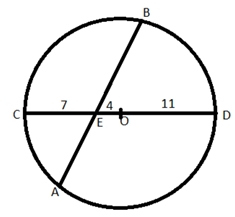In figure AE*BE = CE*DE    (The intersecting chords theorem)
=> 7*15= x(20.5-x)               (Assuming AE = x)
=> 210 = x(41 - 2x)
=> 2x2 - 41x + 210 = 0
=> x = 10 or x = 10.5   => AE = 10 or AE = 10.5  Hence BE = 20.5 - 10 = 10.5
or BE = 20.5 - 10.5 = 10
Required difference = 10.5 -10 = 0.5

Q.23. On selling a pen at 5% loss and a book at 15% gain, Karim gains Rs. 7. If he sells the pen at 5% gain and the book at 10% gain, he gains Rs. 13. What is the cost price of the book in Rupees?
(a) 95
(b) 85
(c) 80
(d) 100
Ans: c
Solution: Assuming the cost price of pen = 100p and the cost price of book = 100b
So, on selling a pen at 5% loss and a book at 15% gain, net gain = -5p + 15b = 7 ....1
On selling the pen at 5% gain and the book at 10% gain, net gain = 5p + 10b = 13  .....2
Adding 1 and 2 we get, 25b = 20
Hence 100b = 20*4 = 80,

Q.24. A chemist mixes two liquids 1 and 2. One litre of liquid 1 weighs 1 kg and one litre of liquid 2 weighs 800 gm. If half litre of the mixture weighs 480 gm, then the percentage of liquid 1 in the mixture, in terms of volume, is
(a) 80
(b) 70
(c) 85
(d) 75
Ans: a
Solution: The weight/volume(g/L) for liquid 1 = 1000
The weight/volume(g/L) for liquid 2 = 800
The weight/volume(g/L) of the mixture = 480/(1/2) = 960
Using alligation the ratio of liquid 1 and liquid 2 in the mixture = (960-800)/(1000-960) = 160/40 = 4 : 1
Hence the percentage of liquid 1 in the mixture = 4*100/(4 + 1) = 80

Q.25. Ramesh and Gautam are among 22 students who write an examination. Ramesh scores 82.5. The average score of the 21 students other than Gautam is 62. The average score of all the 22 students is one more than the average score of the 21 students other than Ramesh. The score of Gautam is
(a) 53
(b) 51
(c) 48
(d) 49
Ans: b
Solution: Assume the average of 21 students other than Ramesh = a
Sum of the scores of 21 students other than Ramesh = 21a
Hence the average of 22 students = a + 1
Sum of the scores of all 22 students = 22(a + 1)
The score of Ramesh = Sum of scores of all 22 students - Sum of the scores of 21 students other than Ramesh = 22(a + 1)-21a = a + 22  = 82.5   (Given)
=> a = 60.5
Hence, sum of the scores of all 22 students = 22(a + 1) = 22*61.5 = 1353
Now the sum of the scores of students other than Gautam = 21*62 = 1302
Hence the score of Gautam = 1353 - 1302 = 51

Q.26. If m and n are integers such that (√2) 1934429m8= 3n16m(∜64) then m is
A -20
B -24
C -12
D -16
Ans: -12
Solution: We have, (√2) 1934429m8n = 3n16m(∜64)
Converting both sides in powers of 2 and 3, we get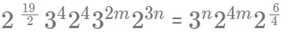Comparing the power of 2 we get,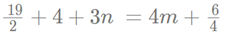=> 4m = 3n + 12 .....(1)
Comparing the power of 3 we get, 4 + 2m = n
Substituting the value of n in (1), we get
4m = 3(4 + 2m) + 12
=> m = -12

Q.27. Three men and eight machines can finish a job in half the time taken by three machines and eight men to finish the same job. If two machines can finish the job in 13 days, then how many men can finish the job in 13 days?
Ans: 13
Solution: Consider the work done by a man in a day = a and that by a machine = b
Since, three men and eight machines can finish a job in half the time taken by three machines and eight men to finish the same job, hence the efficiency will be double.
=> 3a + 8b = 2(3b + 8a)
=> 13a = 2b
Hence work done by 13 men in a day = work done by 2 machines in a day.
=> If two machines can finish the job in 13 days, then same work will be done 13 men in 13 days.
Hence the required number of men = 13

Q.28. Corners are cut off from an equilateral triangle T to produce a regular hexagon H. Then, the ratio of the area of H to the area of T is
(a) 2 : 3
(b) 4 : 5
(c) 5 : 6
(d) 3 : 4
Ans: a
Solution: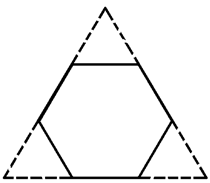The given figure can be divided into 9 regions or equilateral triangles of equal areas as shown below,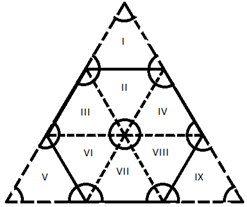Now the hexagon consists of 6 regions and the triangle consists of 9 regions.
Hence the ratio of areas = 6/9 =2 : 3

Q.29. Let x and y be positive real numbers such that log5 (x + y) log5 (x - y) = 3, and log2y - log2x = 1 - log2 3. Then xy equals
(a) 150
(b) 25
(c) 100
(d) 250
Ans: a
Solution: We have, log5 (x + y) log5 (x - y) = 3
=> x2 - y2 = 125.....(1)
log2y - log2x = 1 - log2 3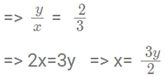On substituting the value of x in 1, we get
5x2/4 = 125
=> y = 10, x = 15
Hence xy = 150

Q.30. Let S be the set of all points (x, y) in the x - y plane such that |x| + |y| ≤ 2 and |x| ≥ 1. Then, the area, in square units, of the region represented by S equals
Ans: 2
Solution: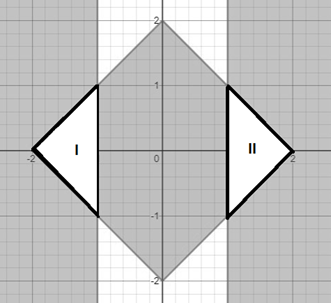Sum of the area of region I and II is the required area.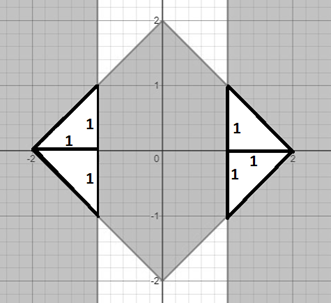Now, required area = 4 x 1/2 x 1 x 1 = 2

Q.31. With rectangular axes of coordinates, the number of paths from (1, 1) to (8, 10) via (4, 6), where each step from any point (x, y) is either to (x, y+1) or to (x+1, y), is
Ans: 3920
Solution: The number of paths from (1, 1) to (8, 10) via (4, 6) = The number of paths from (1, 1) to (4, 6) * The number of paths from (4, 6) to (8, 10)
To calculate the number of paths from (1, 1) to (4, 6), 4 - 1 = 3 steps in x-directions and 6 - 1 = 5 steps in y direction
Hence the number of paths from (1, 1) to (4, 6) = (3 + 5) C3 = 56
To calculate the number of paths from (4, 6) to (8, 10), 8 - 4 = 4 steps in x-directions and 10 - 6 = 4 steps in y direction
Hence the number of paths from (4, 6) to (8, 10) = (4 + 4) C4 = 70
The number of paths from (1, 1) to (8, 10) via (4, 6) = 56*70 = 3920

Q.32. If the rectangular faces of a brick have their diagonals in the ratio 3 : 2 √3 : √15, then the ratio of the length of the shortest edge of the brick to that of its longest edge is
(a) √3 : 2
(b) 1 : √3
(c) 2 : √5
(d) √2 : √3
Ans: b
Solution: Assuming the dimensions of the brick are a, b and c and the diagonals are 3, 2 √3 and √15
Hence, a2 + b2 = 32.....(1)
b2 + c2 = (2√3)2.....(2)
c2 + a2 = (√15)2......(3)
Adding the three equations, 2(a2 + b2 + c2) = 9 + 12 + 15 = 36
=> a2 + b2 + c2 = 18... (4)
Subtracting (1) from (4), we get c2 = 9 => c = 3
Subtracting (2) from (4), we get a2 = 6 => a = √6
Subtracting (3) from (4), we get b2 = 3 => b = √3
The ratio of the length of the shortest edge of the brick to that of its longest edge is = √3/3 = 1 : √3

Q.33. The number of solutions to the equation |x| (6x2 + 1) = 5x2 is
Ans: 5
Solution: For x <0, -x(6x2 + 1) = 5x2
=> (6x2 + 1) = -5x
=> (6x2 + 5x + 1) = 0
=> (6x2 + 3x + 2x + 1) = 0
=> (3x+1)(2x+1) = 0 => x = -1/3 or x = -1/2
For x = 0, LHS = RHS = 0    (Hence, 1 solution)
For x >0, x(6x2 + 1) = 5x2
=> (6x2 - 5x + 1) = 0
=> (3x - 1)(2x - 1) = 0
=> x = 1/3 or x = 1/2
Hence, the total number of solutions = 5

Q.34. Let T be the triangle formed by the straight line 3x + 5y - 45 = 0 and the coordinate axes. Let the circumcircle of T have radius of length L, measured in the same unit as the coordinate axes. Then, the integer closest to L is
Ans: 9
Solution: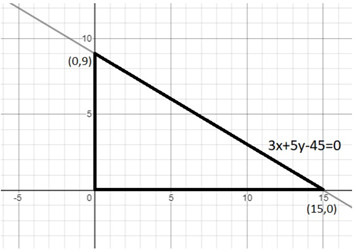In any right triangle, the circumradius is half of the hypotenuse. Here, L = 1/2 * the length of the hypotenuse =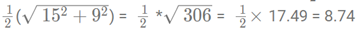Hence, the integer close to L = 9

Offer running on EduRev: Apply code STAYHOME200 to get INR 200 off on our premium plan EduRev Infinity!

,

,

,

,

,

,

,

,

,

,

,

,

,

,

,

,

,

,

,

,

,

;